Innovation for Every Engineer.
HomeBlog

# Comparisons of Resistor in Series and in Parallel

Catalog

 Ⅰ Introduction Ⅱ Resistor network  in series vs in parallel2.1 Resistor in series2.2 Resistor in parallel Ⅲ Resistor Circuit in series vs in parallel3.1 Resistor Circuit in series3.2 Resistor Circuit in parallel Ⅳ Equation in Series and Parallel Ⅴ Examples5.1 Resistors in Series Example5.2 Resistors in Parallel Ⅵ Applications Ⅶ Summary

## Ⅰ Introduction

Individual resistors can be commonly connected to three types of circuits such as series, parallel, or a combination of series and parallel connections to form more complex resistor networks, the equivalent resistance of which is the mathematical combination of the individual resistors connected together.

A resistor is not only a fundmental electronic component that can be applied to convert a voltage to a current or a current to a voltage but it can also be used to place a different weighting on the converted current and/or voltage by correctly adjusting its value, allowing it to be used in voltage reference circuits and applications.

A single equivalent resistor can take place of resistors in series or complicated resistor networks. REQ, or impedance, ZEQ, and regardless of the resistor network's combination or complexity, all resistors follow the same basic rules defined by Ohm's Law and Kirchhoff's Circuit Laws.

Resistors in Series | Electricity and Circuits | Don't Memorise

## Ⅱ Resistor network  in series vs in parallel

### 2.1 Resistor in series

When resistors are daisy-chained together in a single line, they are connected in "Series." Because there is no other way for the current flowing through the first resistor to go, it has to pass through the second, third, and so on. The current that flows through one resistor should flow through the others as well because it can only take one path, so resistors in series have a Common Current flowing through them.

The current flowing through a series of resistors will then be the same at all points in a series resistor network. As an example:In the following example, resistors R1, R2, and R3 are connected in series between points A and B, with a common current, I, flowing through them.

### 2.2 Resistor in parallel

In contrast to the previous series resistor circuit, the circuit current in a parallel resistor network can take more than one path because there are multiple paths for the current. Parallel circuits are then classified as current dividers.

Because the supply current can flow through multiple paths, the current may not be the same through all of the parallel network's branches. The voltage drop across all resistors in a parallel resistive network, on the other hand, so it is. Then, parallel-connected resistors have a common voltage across them, as do all parallel-connected elements.## Ⅲ Resistor Circuit in series vs in parallel

### 3.1 Resistor Circuit in series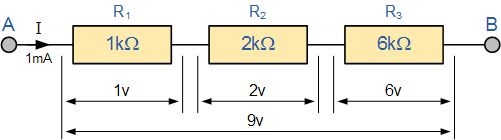Resistor Circuit in series

Because the resistors are linked in series, the same current flows through each resistor in the chain, and the total resistance, RT, of the circuit must equal the sum of all the individual resistors added together. That is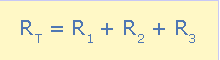and by taking the individual values of the resistors in our simple example above, the total equivalent resistance, REQ is therefore given as:

REQ = R1 + R2 + R3 = 1kΩ + 2kΩ + 6kΩ = 9kΩ

### 3.2 Resistor Circuit in parallel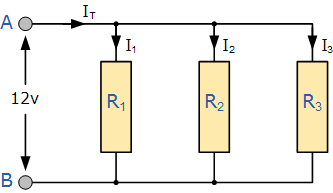The total resistance, RT, of the circuit in the previous series resistor network was equal to the sum of all the individual resistors added together. The equivalent circuit resistance RT is calculated differently for parallel resistors.

Instead of the resistances themselves, the reciprocal (1/R) value of each is added together, with the inverse of the algebraic sum giving the equivalent resistance as shown.

Instead of the resistances themselves, the reciprocal (1/R) value of each is added together, with the inverse of the algebraic sum giving the equivalent resistance as shown.

## Ⅳ Equation in Series and Parallel

### 4.1 Series Resistor Equation

Because it is the algebraic sum of the individual resistances, the total or equivalent resistance, RT, has the same effect on the circuit as the original combination of resistors.

If two equal and of the same value resistances or impedances are connected in series, the total or equivalent resistance, RT, is equal to twice the value of one resistor. That is equal to 2R for two equal resistors in series, 3R for three equal resistors in series, and so on.Series Resistor Equation

If two series resistors or impedances are unequal and of different values, the total or equivalent resistance, RT, is equal to the mathematical sum of the two resistances. R1 + R2 is the answer. The equivalent resistance of three or more unequal (or equal) resistors connected in series is: R1 + R2 + R3 +..., etc.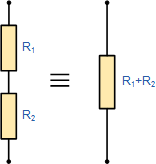One important thing to remember about resistors in series networks is to double-check your math. The total resistance (RT) of any two or more resistors connected in series is always greater than the value of the chain's largest resistor. In our previous example, RT = 9k, whereas the largest resistor value is only 6k.

### 4.2 Parallel Resistor Equation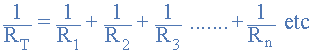The algebraic sum of the inverses of the individual resistances is the inverse of the equivalent resistance of two or more resistors connected in parallel.

If the two parallel resistances or impedances are equal and of the same value, the total or equivalent resistance, RT, is equal to half the value of one resistor. That is R/2 for two equal resistors in parallel, R/3 for three equal resistors in parallel, and so on.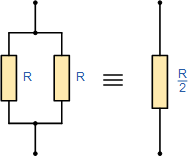Because the equivalent resistance is always less than the smallest resistor in the parallel network, as more parallel resistors are added, the total resistance, RT, will always decrease.

## Ⅴ Examples

### 5.1 Resistors in Series Example

• Calculate the voltage drops across X and Y
1. a) Without RL connected

1. b) With RL connectedseries example

As shown above, the output voltage Vout without the load resistor connected gives us the required output voltage of 6V, but when the load is connected, the output voltage drops to only 4V. (Resistors in Parallel).

Then we can see that a loaded voltage divider network's output voltage changes as a result of the loading effect because the output voltage Vout is determined by the R1 to R2 ratio. However, as the load resistance, RL, approaches infinity (), the loading effect diminishes and the voltage ratio of Vout/Vs is unaffected by the addition of the load on the output. Then, as the load impedance increases, the loading effect on the output decreases.

Attenuation is the effect of lowering a signal or voltage level, so when using a voltage divider network, it is essential to have cautiousness. This loading effect could be compensated for by using a potentiometer instead of fixed value resistors and adjusting the potentiometer accordingly. This method also compensates the potential divider for variations in resistor tolerances.

### 5.2 Resistors in Parallel

Find the total resistance, RT of the following resistors connected in a parallel network.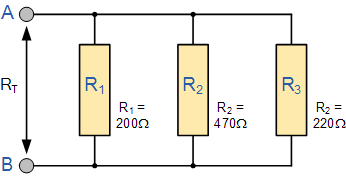The total resistance RT across the two terminals A and B is calculated as: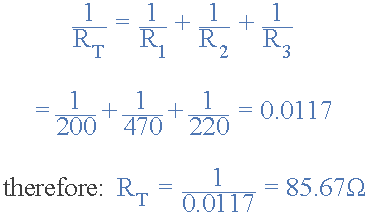This reciprocal calculation method can be used to calculate any number of individual resistances connected in a single parallel network.

If, on the other hand, there are only two individual resistors connected in parallel, we can use a much simpler and faster formula to find the total or equivalent resistance value, RT, and thus help reduce the reciprocal maths a little.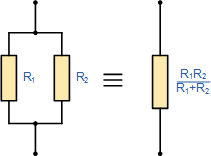## Ⅵ Applications

We've seen how Resistors in Series can be applied to generate different voltages across themselves, and how this genre of resistor network can be used to create a voltage divider network. We can convert an analog quantity being sensed into a suitable electrical signal that can be measured by replacing one of the resistors in the voltage divider circuit above with a Sensor such as a thermistor, light-dependent resistor (LDR), or even a switch.

The following thermistor circuit, for example, has a resistance of 10K at 25°C and a resistance of 100 at 100°C. Determine the output voltage (Vout) for each temperature.

### 6.2 Various Parallel Resistor Networks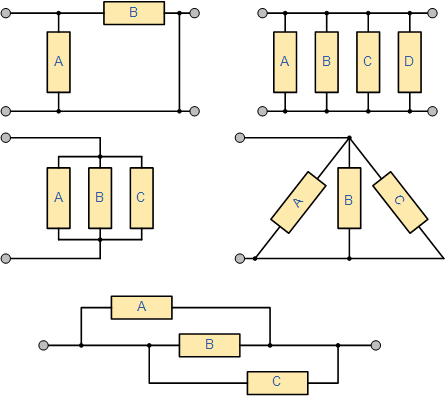The five resistive networks shown above may appear to be different, but they are all arranged as Resistors in Parallel, and thus the same conditions and equations apply.

## Ⅶ Summary

### 7.1 Resistors in Series Summary

When two or more resistors are connected end-to-end in a single branch, Reputedly, they are connected in series. Resistors in series carry the same current, but the voltage drop across them is not the same as their resistance values result in different voltage drops across each resistor, as determined by Ohm's Law (V = I*R). Then there are series circuits, which are voltage dividers.

Individual resistors in a series resistor network add together to give the series combination's equivalent resistance, (RT). A series circuit's resistors can be swapped without affecting the total resistance, current, or power to each resistor or the circuit.

### 7.2 Resistors in Series parallel

When two or more resistors are connected in such a way that their terminals are connected to the terminals of the other resistor or resistors, they are connected in parallel. The voltage across each resistor in a parallel combination is the same, but the currents flowing through them are not because of their resistance value and Ohms Law. Parallel circuits are then used as current dividers.

Reciprocal addition is used to find the equivalent or total resistance, RT, of a parallel combination, and the total resistance value is always less than the smallest individual resistor in the combination. Within the same combination, parallel resistor networks can be swapped without changing the total resistance or total circuit current. Resistors connected in a parallel circuit will continue to operate even if one of them is open-circuited.